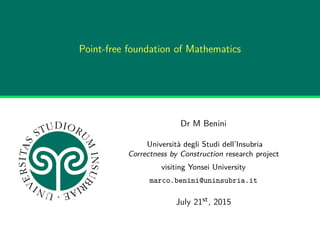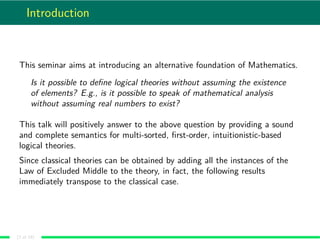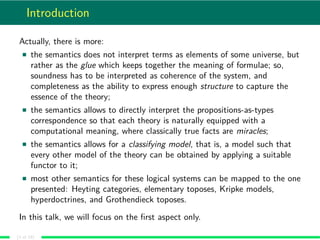Successfully reported this slideshow.

# Point-free foundation of Mathematics

1

Share×
1 of 18
1 of 18

# Point-free foundation of Mathematics

1

Share

A talk I gave at the Yonsei University, Seoul in July 21st, 2015.
The aim was to show my background contribution to the CORCON (Correctness by Construction) research project.
I have to thank Prof. Byunghan Kim and Dr Gyesik Lee for their kind hospitality.

A talk I gave at the Yonsei University, Seoul in July 21st, 2015.
The aim was to show my background contribution to the CORCON (Correctness by Construction) research project.
I have to thank Prof. Byunghan Kim and Dr Gyesik Lee for their kind hospitality.

## More Related Content

### Related Books

Free with a 14 day trial from Scribd

See all

### Related Audiobooks

Free with a 14 day trial from Scribd

See all

### Point-free foundation of Mathematics

1. 1. Point-free foundation of Mathematics Dr M Benini Università degli Studi dell’Insubria Correctness by Construction research project visiting Yonsei University marco.benini@uninsubria.it July 21st, 2015
2. 2. Introduction This seminar aims at introducing an alternative foundation of Mathematics. Is it possible to deﬁne logical theories without assuming the existence of elements? E.g., is it possible to speak of mathematical analysis without assuming real numbers to exist? This talk will positively answer to the above question by providing a sound and complete semantics for multi-sorted, ﬁrst-order, intuitionistic-based logical theories. Since classical theories can be obtained by adding all the instances of the Law of Excluded Middle to the theory, in fact, the following results immediately transpose to the classical case. (2 of 18)
3. 3. Introduction Actually, there is more: the semantics does not interpret terms as elements of some universe, but rather as the glue which keeps together the meaning of formulae; so, soundness has to be interpreted as coherence of the system, and completeness as the ability to express enough structure to capture the essence of the theory; the semantics allows to directly interpret the propositions-as-types correspondence so that each theory is naturally equipped with a computational meaning, where classically true facts are miracles; the semantics allows for a classifying model, that is, a model such that every other model of the theory can be obtained by applying a suitable functor to it; most other semantics for these logical systems can be mapped to the one presented: Heyting categories, elementary toposes, Kripke models, hyperdoctrines, and Grothendieck toposes. In this talk, we will focus on the ﬁrst aspect only. (3 of 18)
4. 4. Introduction Most of this talk is devoted to introduce a single deﬁnition, logical categories, which identiﬁes the models of our logical systems. These models are suitable categories, equipped with an interpretation of formulae and a number of requirements on their structure. Although the propositional part has already been studied by, e.g., Paul Taylor, the ﬁrst-order extension is novel. (4 of 18)
5. 5. Logical categories Let 〈S,F,R〉 be a ﬁrst-order signature, with S the set of sort symbols, F the set of function symbols, of the form f : s1 ×···×sn → s0, with si ∈ S for all 0 ≤ i ≤ n, R the set of relation symbols, of the form r : s1 ×···×sn, with si ∈ S for all 1 ≤ i ≤ n. Also, let T be a theory on 〈S,F,R〉, i.e., a collection of axioms. A logical category will be a pair 〈C,Σ〉 where C is a suitable category and Σ a family of functors modelling the substitution of terms in interpreted formulae. Informally, the objects of C will denote formulae while arrows will denote proofs where the codomain is the theorem and the domain is the assumption(s). (5 of 18)
6. 6. Logical categories Deﬁnition 1 (Prelogical category) Fixed a language where T is the discrete category of its terms, a prelogical category is a pair 〈C,Σ〉 such that 1. C is a category with ﬁnite products, ﬁnite coproducts and exponentiation; 2. for each formula A and each variable x :s, ΣA(x :s): T → C is a functor, called the substitution functor, such that, for every variable y :s , ΣA(x :s)(x :s) = ΣA(y :s )(y :s ); 3. for each x :s variable, t :s term, A and B formulae, 3.1 Σ⊥(x :s)(t :s) = 0, the initial object in C; 3.2 Σ (x :s)(t :s) = 1, the terminal object in C; 3.3 ΣA∧B(x :s)(t :s) = ΣA(x :s)(t :s)×ΣB(x :s)(t :s), the binary product in C; 3.4 ΣA∨B(x :s)(t :s) = ΣA(x :s)(t :s)+ΣB(x :s)(t :s), the binary coproduct in C; 3.5 ΣA⊃B(x :s)(t :s) = ΣB(x :s)(t :s)ΣA(x :s)(t :s), the exponential object in C; 4. for any formula A, any variable x :s, and any term t :s, ΣA(x :s)(t :s) = ΣA[t/x](x :s)(x :s). (6 of 18)
7. 7. Logical categories The substitution functors model the operation of substituting a variable with a term in an interpreted formula. This behaviour is ﬁxed by the fourth condition: it says how the syntactical act of substituting a term for a variable in formula is performed within the semantics. These functors allow to deﬁne the notion of interpretation, associating every formula A to an object in the category: MA = ΣA(x :s)(x :s). This deﬁnition is well-founded, thanks to the second condition. So propositional intuitionistic logic is readily interpreted, as shown in P. Taylor, Practical foundations of mathematics, Cambridge University Press, 1999. Of course, the problem lies in quantiﬁed formulae. . . (7 of 18)
8. 8. Logical categories A star S over a formula A and a variable x :s is a subcategory of C such that: 1. its objects are the vertexes v of the cones on ΣA(x :s) such that v = MB for some formula B and x :s ∈ FV(B), i.e., x is not free in B; 2. there is an object MC in S, its centre, such that all the morphisms in S are either identities, or arrows in the category of cones on ΣA(x :s) with MC as codomain. MB µ // θt ** MC πttt ΣX (x)(t) t∈T Dually, one may deﬁne the notion of costar. Intuitively, the centre of a star is the natural candidate to interpret the formula ∀x :s.A, with the arrows in S standing for instances of the introduction rule, while the projections in the cone whose vertex is the centre, denote instances of the elimination rule. Dually, costars are used to provide a meaning to ∃x :s.A. (8 of 18)
9. 9. Logical categories Deﬁnition 2 (Logical category) A prelogical category 〈C,Σ〉 is called logical when, for each formula A and each variable x :s, 1. there is a star on A and x :s, denoted by C∀x :s.A, having a terminal object; we require M∀x :s.A to be the centre of C∀x :s.A; 2. there is a costar on A and x :s, denoted by C∃x :s.A, having an initial object; we require M∃x :s.A to be the centre of C∃x :s.A; It is possible to prove that each formula in any theory T in the ﬁrst-order language can be interpreted in this class of categories. Also, any proof can be naturally represented by a morphism in this class of categories. This interpretation is both sound and complete: we say that a formula is true when there is an arrow from 1 to its interpretation. Thus, a model for a theory T is such that it makes true any axiom in T. Then, every provable formula in a theory T is true in every model of T. And, vice versa, any formula which is true in any model for T, is provable from T. (9 of 18)
10. 10. Logical categories Soundness is not surprising: since arrows denote proofs, each arrow which is required by the structure, denote an inference rule. For example, the projections of the product denote the ∧-elimination rules, while the universal arrow of the product is the ∧-introduction rule. Completeness proceeds by showing that the syntactical category over a theory T, having formulae-in-context as objects and proofs as arrows (well, modulo an equivalence relation that takes care of multiple assumptions, like in P. Johnstone’s Sketches of an Elephant), is a logical category which is also a model for T. Moreover, this model is a classifying model: any other model for T can be derived by applying to the syntactical category a suitable logical functor, i.e., a functor that preserves ﬁnite limits and ﬁnite colimits. These proofs are long, complex, and quite technical. Too boring for a talk. . . (10 of 18)
11. 11. The role of terms How terms get interpreted? Variables are used to identify the required subcategories C∀x : s.A and C∃x : s.A; variables are also used to apply the ΣA(x :s) functor properly; all terms contribute to the substitution process, which induces the structure used by the semantics. Thus, it is really the substitution process, formalised in the ΣA(x :s) functors, that matters: terms are just the glue that enable us to construct the C∀x : s.A and C∃x : s.A subcategories. It is clear the topological inspiration of the whole construction. In particular, it is evident that terms are not interpreted in some universe, and their role is limited to link together formulae in subcategories that control how quantiﬁers are interpreted. (11 of 18)
12. 12. Abstract syntax There is no need to consider the set of terms as the only way to provide a language for the “elements” of the theory: any groupoid will do. Since in a groupoid G, all the arrows are isomorphisms, the substitution functor forces them to become instances of the substitution by equals principle. Variables are not needed, as well: the notion of variable can be deﬁned by posing that x ∈ ObjG is a variable when there is a formula A and an object t ∈ ObjG for which the substitution functor ΣA(x)(t) = MA, i.e., a variable is something which may be substituted obtaining something diﬀerent. Also a variable is free in a formula when it may be substituted in the formula to obtain a diﬀerent one. When the variables in a groupoid G are inﬁnite, or there are none, the purely propositional case, the soundness and completeness results can be proven as before. Also, ﬁxed a logical category C, we can regard its objects as abstract formulae, with no harm in the above approach to semantics: in this case, C becomes the classifying model for the theory whose language is given by itself, and whose axioms are its true formulae. (12 of 18)
13. 13. Inconsistent theories A theory T is inconsistent when it allows to derive falsity. However, in our semantics, T has a model as well. A closer look to each model of T reveals that they are categorically “trivial” in the sense that the initial and the terminal objects are isomorphic. This provides a way to show that a theory is consistent. However, this is not ultimately easier or diﬀerent than ﬁnding an internal contradiction in the theory. Actually, it does make sense in a purely computational view that an inconsistent theory has a model: it means that, although the speciﬁcation of a program is ultimately wrong, there are pieces of code which are perfectly sound. And every computation does something, even if not useful! (13 of 18)
14. 14. Alternative semantics Most semantics for ﬁrst-order intuitionistic logic and theories can be reduced to elementary toposes via suitable mappings. This is the case for Heyting categories, for Grothendieck toposes, and, in an abstract sense, with hyperdoctrines. Since algebraic semantics and Kripke semantics can be reduced to Heyting categories and Grothendieck toposes, respectively, we can safely say that elementary toposes provide a good framework to compare semantics. It is possible to show that any model in any elementary topos can be transformed in a model M in a logical category in such a way that exactly the true formulae in the topos are true in the M. The converse does not hold in a direct way: in fact, assuming to work in a strong set theory, one can show that a topos model can be reconstructed from M by means of a Kan extension. The diﬃcult part is that one should assume enough power in the set theory to literally build up a collection of elements big enough to contain the interpretations of terms and of every other element which is implied to exist by the theory. (14 of 18)
15. 15. State of the Art At the moment, these results have been submitted for a possible publication. Also, they have been presented in a few workshops and in a number of seminars within the CORCON project. My current research on this topic follows three main lines: 1. to extend the idea beyond ﬁrst-order systems. Speciﬁcally, I am trying to provide a point-free semantics to constructive type theory, with a look towards homotopy type theory. This work has made some progress, but the results are not yet obtained and not everything is stable. 2. to apply these results to concrete theories. In this respect, I am developing a presentation of the theory of well quasi-orders along these lines, using as a logical category the category of quasi-orders, coupled with substitution functors acting on singletons as variables, and ﬁnite chains as terms. Some results have been obtained, and they will be presented in an upcoming workshop in Hamburg, Germany, in September. 3. to clean up the framework, to understand exactly how much power is required to get the results, and to ﬁnd the minimal fragment of category theory/set theory required to develop the point-free semantics. (15 of 18)
16. 16. Projects This work has been supported by two research projects: Abstract Mathematics for Actual Computation: Hilbert’s Program in the 21st Century, 2014-2016, John Templeton Foundation, Core Funding. Partner: University of Leeds (UK) Correctness by Construction, 2014-2017, FP7-PEOPLE-2013-IRSES, gr.n. PIRSES-GA-2013-612638. Partner: University of Leeds (UK), University of Strathclyde (UK), Swansea University (UK), Stockholms Universitet (SE), Universitaet Siegen (DE), Ludwig-Maximilians Universitaet Muenchen (DE), Università degli Studi di Padova (IT), Università degli Studi di Genova (IT), Japan Advanced Institute of Science and Technology (JP), University of Canterbury (NZ), The Australian National University (AU), Institute of Mathematical Sciences (IN), Carnegie Mellon University (US), Hankyong National University (KR), Kyoto University (JP), National Institute of Informatics (JP), Tohoku University (JP), University of Gothenburg (SE), University of Ljubljana (SI) (16 of 18)
17. 17. My visit in Korea Since Dr Lee is a partner in the CORCON project, my visit is the chance to share the achievement we have made. The next theory to develop in point-free terms will be real analysis: in this respect, I will try to propose a research project to the European Union next year. It will be a joint eﬀort with numerical analysts, starting from the very simple idea that a real number in numerical analysis is actually a point-free entity, denoted by its value and its error. A clean and neat logical framework to deal with this idea in a direct way may be of beneﬁt both for logicians, since it explains how numerical computations on reals are designed, and for numerical analysts, since it is much closer to the way they have to think when solving problems numerically. (17 of 18)
18. 18. Conclusion Much more has been done on logical categories. But, still, I am in the beginning of the exploration of this foundational setting. So, any comment or suggestion is welcome! Questions? (18 of 18)